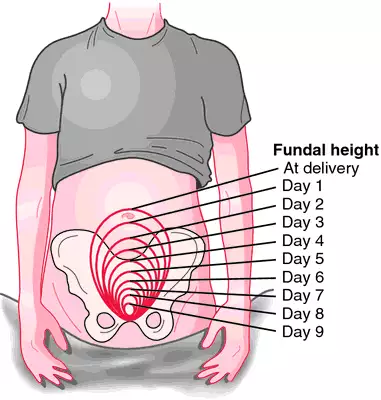# involution

(redirected from involutions)
Also found in: Dictionary, Thesaurus, Encyclopedia.

## involution

[in″vo-lu´shun]
1. a rolling or turning inward.
2. one of the movements involved in the gastrulation of many animals.
3. a retrograde change of the entire body or in a particular organ, as the retrograde changes in the female genital organs that result in normal size after delivery.
4. the progressive degeneration occurring naturally with advancing age, resulting in shriveling of organs or tissues. adj., adj involu´tional.Involution of the uterus. Height of the uterine fundus decreases by approximately 1 cm/day. From McKinney et al., 2000.

## in·vo·lu·tion

(in'vō-lū'shŭn),
1. Return of an enlarged organ to normal size.
2. Turning inward of the edges of a part.
3. In psychiatry, mental decline associated with advanced age.
Synonym(s): catagenesis
[L. in-volvo, pp. -volutus, to roll up]

## involution

(ĭn′və-lo͞o′shən)
n.
1.
a. The act of involving.
b. The state of being involved.
2. Intricacy; complexity.
3. Something, such as a long grammatical construction, that is intricate or complex.
4. Mathematics An operation, such as negation, which, when applied to itself, returns the original number.
5. Embryology The ingrowth and curling inward of a group of cells, as in the formation of a gastrula from a blastula.
6. Medicine
a. A decrease in size of an organ, as of the uterus following childbirth.
b. A progressive decline or degeneration of normal physiological functioning occurring as a result of the aging process.

## involution

Gynecology See Uterine involvement Medtalk A ↓in organ size or functional capacity, generally understood to be age-related.

## in·vo·lu·tion

(in'vŏ-lū'shŭn)
1. Return of an enlarged organ to normal size.
2. Turning inward of the edges of a part.
3. psychiatry Mental decline associated with advanced age.
Synonym(s): catagenesis.
[L. in-volvo, pp. -volutus, to roll up]

## involution

1. Decay, retrogression or shrinkage in size.
3. An infolding or INVAGINATION.

## involution

(of plant organs) having rolled-up margins.

## in·vo·lu·tion

(in'vŏ-lū'shŭn)
1. Return of an enlarged organ to normal size.
2. Turning inward of the edges of a part.
[L. in-volvo, pp. -volutus, to roll up]
References in periodicals archive ?
In this paper we shall use a spectral problem for an ordinary differential operators with involution. Such and similar spectral problems were considered in [29-40].
Involutions fixing the disjoint union of many projective 2r + 1-spaces, Northeast.
Proof: This is immediate from Corollary 5.3, since the number of 3412-avoiding involutions of length [l.sub.j] - 1 is [MATHEMATICAL EXPRESSION NOT REPRODUCIBLE IN ASCII] for 1 [less than or equal to] j [less than or equal to] m.
Lemma 2 Let F be a set of fixed point free involutions which is expanded and closed under deflation; let G be the set of its irreducible involutions.
After parturition, suitable approach is proposed to administer oxytocin for three days followed by prostaglandins for three days for better expulsion of placenta and its shreds with speedy and early uterine involution. Oxytocin @ 30-50 IU and prostaglandin @ 25 mg can be used.
If [iota] is an r-admissible involution of [Z.sub.F], we denote by [F.sub.[iota]] the set of symbols [MATHEMATICAL EXPRESSION NOT REPRODUCIBLE IN ASCII] in F such that [absolute value of ([beta] [intersection [omega])] = 1 for all [iota]-orbits [omega].
In particular, if at least one of the groups A, B modulo scalars has even order then repeated applications of IJ may construct an element belonging to A or B .In the implementation, we add powers of 10 random elements to L, and the results of 10 applications of the involution jumper.
[not equal to] 2, D a division k-algebra with center K = k([square root of (a)]) and involution J of second kind, non-trivial on K and h a non-degenerate hermitian form of rank n with respect to J and with values in D.
Similar involutions were studied by Kirillov and Berenstein (1995) in the context of Gelfand-Tsetlin triangles.
In this paper we study the polynomial [S.sub.n](x) = [[summation].sup.n-1.sub.j=0] [s.sub.n,j] [x.sup.j], where [s.sub.n,j] denotes the number of centrosymmetric involutions on [n] with j ascents (and hence n - 1 - j descents).
Here the clawlike hardware gripping the stacked sequence of leather cylinders also acts to hold open their outer layer to bare the alarmingly raw and flesh-like involutions of the subcutaneous interior.
They cover Moufang quadrangles, residues in Bruhat-Tits buildings, descent, Galois involutions, and exceptional Tits indices.

Site: Follow: Share:
Open / Close